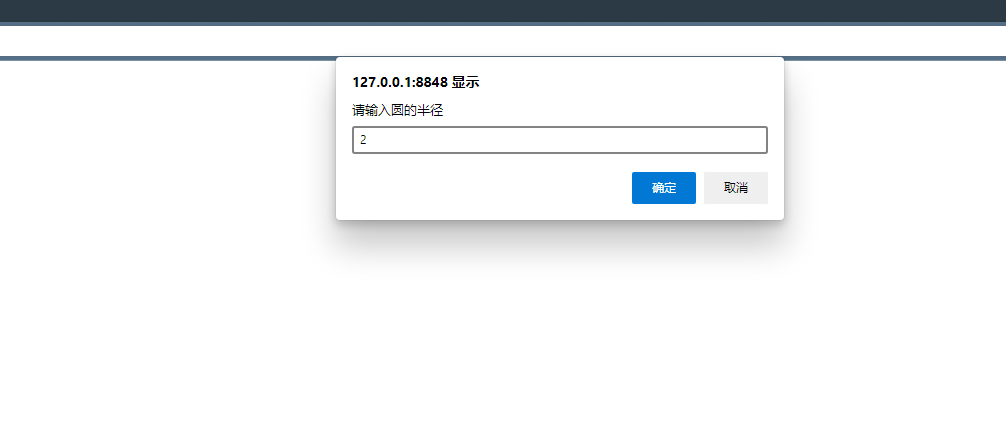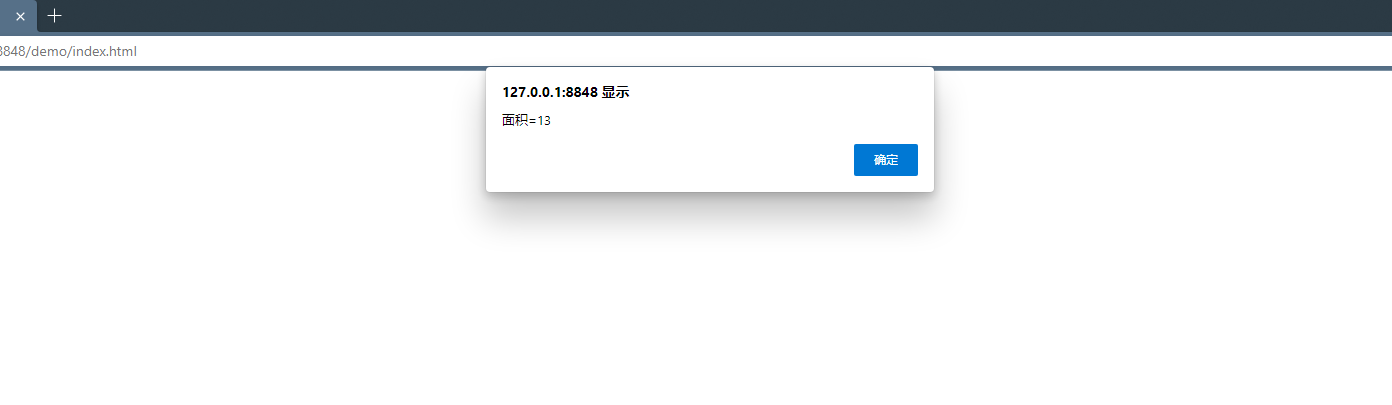javascript求圆面积的代码是什么

Math 对象并不像 Date 和 String 那样是对象的类，因此没有构造函数 Math()。

var x = Math.PI; // 返回PI
var y = Math.sqrt(16); // 返回16的平方根

Math.pow(x,y)

//pow() 方法返回 x 的 y 次幂。

<!DOCTYPE html>
<html>
<meta charset="utf-8">
<title>javascript求圆面积的代码是什么</title>
<body>
<div>

</div>
<script type="text/javascript">
function round(number, X) {
X = (!X ? 2 : X);
return Math.round(number*Math.pow(10,X)/Math.pow(10,X));
}
var r = Number(prompt('请输入圆的半径'));
if(!isNaN(r)) {
var m = round(Math.PI * Math.pow(r, 2), 2);
}else{
}
</script>
</body>
</html>javascript怎样判断奇偶数

2021-12-10 20:06:30

测试

2021-12-22 17:43:38

0 条回复 A文章作者 M管理员
暂无讨论，说说你的看法吧